﻿

# 複雜之投資報酬率

### 一般坊間算法

200,000經過12個月的複利得到：200000*(1 + r / 12)12
110,000經過 6個月的複利得到：110000*(1 + r / 12)6

200000*(1 + r / 12)12 + 110000*(1 + r / 12)6 = 360000 --------------------------- (1)

200000*(1+1.51687%)12 + 110000*(1+1.51687%)6 = 239,601 + 120,399 = 360,000
Excel公式 =200000*(1+1.51687%)^12 + 110000*(1+1.51687%)^6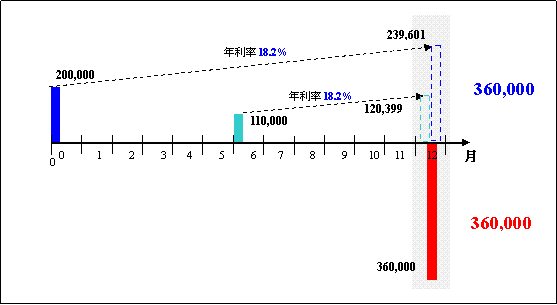200000*(1 + r / 12)12 + 110000*(1 + r / 12)6 = 360000 + 6000*(1+r/12)9----------- (2)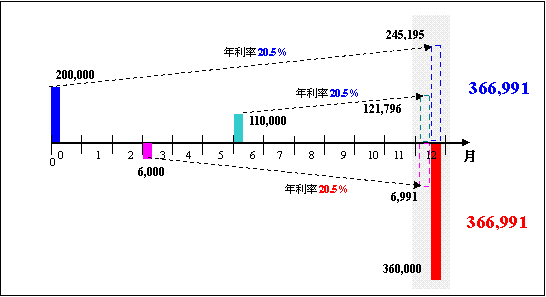200000*(1 + 20.55% / 12)12 = 245,195
110000*(1 + 20.55% / 12)6 = 121,796
6000*(1 + 20.55% / 12)9 = 6,991

245,195 + 121,796 = 6,991+360,000 = 366,991

# 內部報酬率(IRR)# IRR之應用

## 整存整付定存

100萬放銀行整存整付定存，一年後拿回103萬，求銀行牌告年利率

##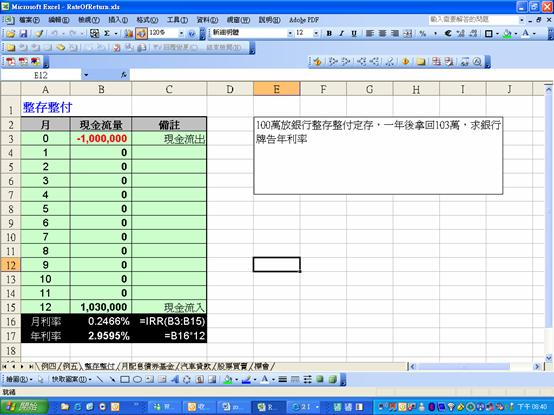月配息債券基金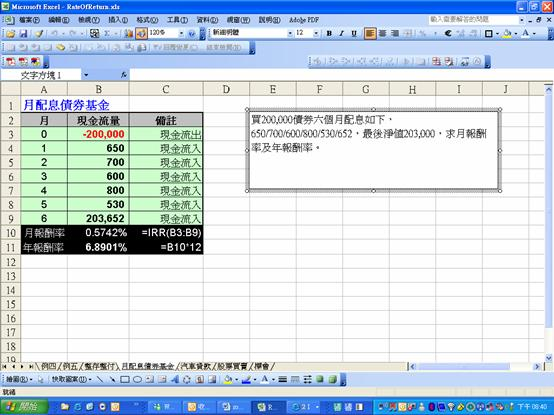## 汽車貸款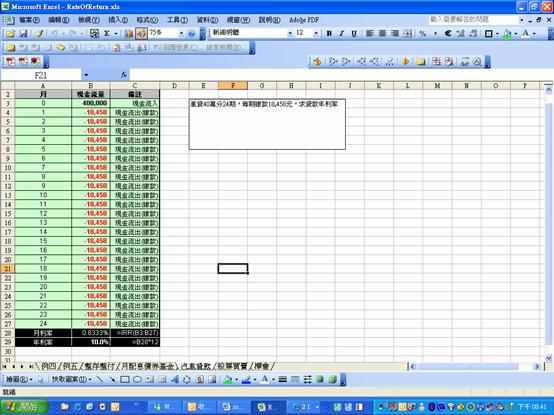## 股票買賣

30元/股買進中鋼10張，第3個月配息2元/股，第5個月以33元/股賣出4張，第8個月以26元/股再買6張攤平，最後於第11個月時以30元賣出所剩12張股票，求該項投資之年報酬率。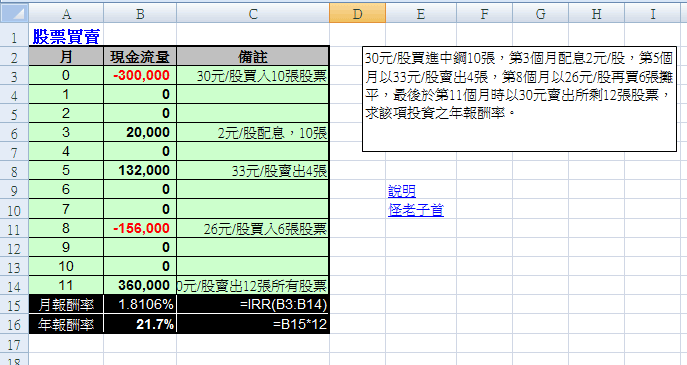## 標會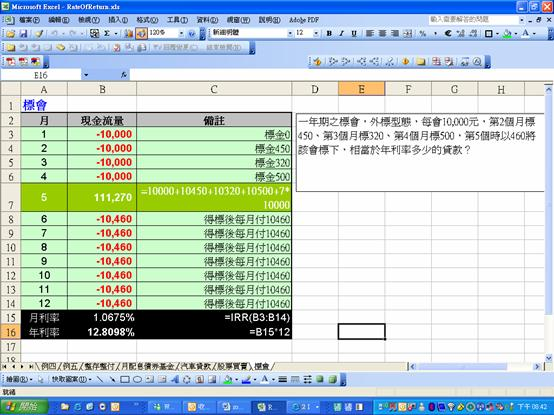# 附註：貨幣的時間價值

 期初本金 利息 期末本利和 第一年 100 10 110 第二年 110 11 121 第三年 121 12.1 133.1 第四年 133.1 13.31 146.41 第五年 146.41 14.641 161.051121 = 110 x 1.1
= (100 x 1.1) x 1.1
= 100 x (1.1 x 1.1)
= 100 x 1.12
= 100 x 1.21

133.1= 121 x 1.1
= (110 x 1.1) x 1.1
= (100 x 1.1) x 1.1 x 1.1
= 100 x 1.13
= 100 x 1.331

FV = PV*(1 + r )t

=100*(1+10%)5 = 161.051

Excel 公式 =100*(1+10%)^5

### 月複利範例

FV = 10000*(1+0.18333%)12 = 10,222

Excel 公式 =10000*(1+0.18333%)^12

### 現值 PV

PV = FV / (1 + r )t

PV = US\$50,000/(1+5%)5 = 39,176 ;
EXCEL公式 =50000/(1+5%)^5

﻿

■ 本網站內容儘可能精確完整，但不保證無誤。若做為投資依據，風險請自行斟酌 ，本網站不負賠償之責任。
■ 網站所有資料均為版權所有，非經書面允許請勿轉載或使用。